Balbharati Maharashtra State Board 12th Commerce Maths Solution Book Pdf Chapter 5 Index Numbers Miscellaneous Exercise 5 Questions and Answers.

## Maharashtra State Board 12th Commerce Maths Solutions Chapter 5 Index Numbers Miscellaneous Exercise 5

(I) Choose the correct alternative.

Question 1.
Price Index Number by Simple Aggregate method is given by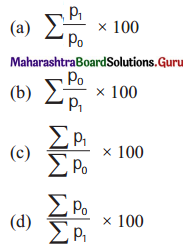(c) $$\frac{\sum p_{1}}{\sum p_{0}} \times 100$$

Question 2.
Quantity Index Number by Simple Aggregate Method is given by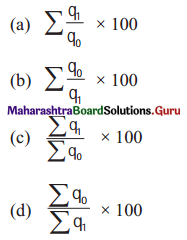(c) $$\frac{\sum q_{1}}{\sum q_{0}} \times 100$$Question 3.
Value Index Number by Simple Aggregate Method is given by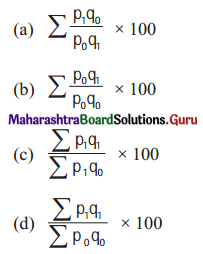(b) $$\sum \frac{p_{0} q_{1}}{p_{0} q_{0}} \times 100$$

Question 4.
Price Index Number by Weighted Aggregate Method is given by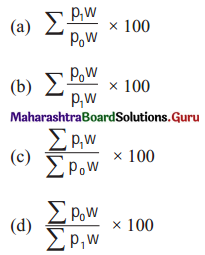(c) $$\frac{\sum p_{1} w}{\sum p_{0} w} \times 100$$

Question 5.
Quantity Index Number By Weighted Aggregate Method is given by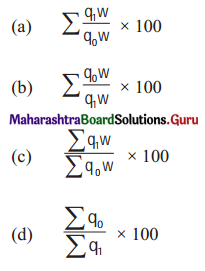(c) $$\frac{\sum q_{1} w}{\sum q_{0} w} \times 100$$

Question 6.
Value Index Number by Weighted aggregate Method is given by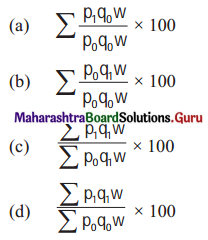(d) $$\frac{\sum p_{1} q_{1} w}{\sum p_{0} q_{0} w} \times 100$$

Question 7.
Laspeyre’s Price Index Number is given by(c) $$\frac{\sum p_{1} q_{0}}{\sum p_{0} q_{0}} \times 100$$Question 8.
Paassche’s Price Index Number is given by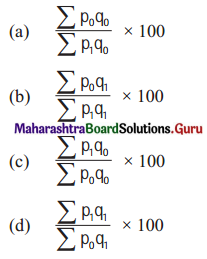(d) $$\frac{\sum p_{1} q_{1}}{\sum p_{0} q_{1}} \times 100$$

Question 9.
Dorbish-Bowley’s Price Index Number is given by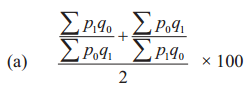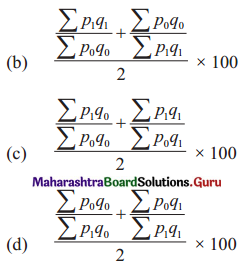(c) $$\frac{\frac{\sum p_{1} q_{0}}{\sum p_{0} q_{0}}+\frac{\sum p_{1} q_{1}}{\sum p_{0} q_{1}}}{2} \times 100$$

Question 10.
Fisher’s Price Number is given by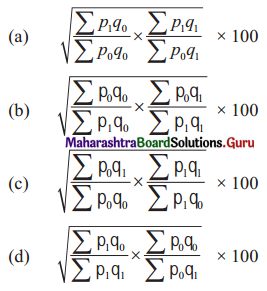(a) $$\sqrt{\frac{\sum p_{1} q_{0}}{\sum p_{0} q_{0}} \times \frac{\sum p_{1} q_{1}}{\sum p_{0} q_{1}}} \times 100$$

Question 11.
Marshall-Edge worth’s Price Index Number is given by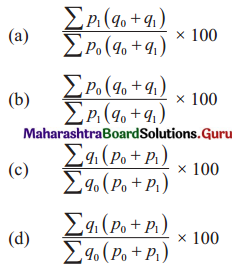(a) $$\frac{\sum p_{1}\left(q_{0}+q_{1}\right)}{\sum p_{0}\left(q_{0}+q_{1}\right)} \times 100$$

Question 12.
Walsh’s Price Index Number is given by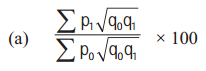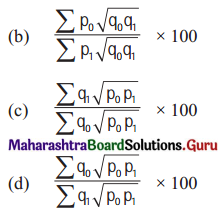(a) $$\frac{\sum p_{1} \sqrt{q_{0} q_{1}}}{\sum p_{0} \sqrt{q_{0} q_{1}}} \times 100$$

Question 13.
The Cost of Living Index Number using Aggregate Expenditure Method is given by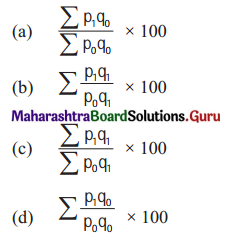(a) $$\frac{\sum p_{1} q_{0}}{\sum p_{0} q_{0}} \times 100$$Question 14.
The Cost of Living Index Number using Weighted Relative Method is given by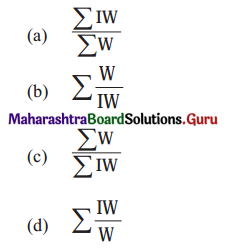(a) $$\frac{\sum \mathrm{IW}}{\sum \mathrm{W}}$$

(II) Fill in the blanks.

Question 1.
Price Index Number by Simple Aggregate Method is given by ____________
$$\frac{\sum p_{1}}{\sum p_{0}} \times 100$$

Question 2.
Quantity Index number by Simple Aggregate Method is given by ____________
$$\frac{\sum q_{1}}{\sum q_{0}} \times 100$$

Question 3.
Value Index Number by Simple Aggregate Method is given by ____________
$$\frac{\sum p_{1} q_{1}}{\sum p_{0} q_{0}} \times 100$$

Question 4.
Price Index Number by Weighted Aggregate Method is given by ____________
$$\frac{\sum p_{1} w}{\sum p_{0} w} \times 100$$

Question 5.
Quantity Index Number by Weighted Aggregate Method is given by ____________
$$\frac{\sum q_{1} w}{\sum q_{0} w} \times 100$$

Question 6.
Value Index Number by Weighted Aggregate Method is given by ____________
$$\frac{\sum p_{1} q_{1} w}{\sum p_{0} q_{0} w} \times 100$$Question 7.
Laspeyre’s Price Index Number is given by ____________
$$\frac{\sum p_{1} q_{0}}{\sum p_{0} q_{0}} \times 100$$

Question 8.
Paasche’s Price Index Number is given by ____________
$$\frac{\sum p_{1} q_{1}}{\sum p_{0} q_{1}} \times 100$$

Question 9.
Dorbish-Bowley’s Price Index Number is given by ____________
$$\frac{1}{2}\left[\frac{\sum p_{1} q_{0}}{\sum p_{0} q_{0}}+\frac{\sum p_{1} q_{1}}{\sum p_{0} q_{1}}\right] \times 100$$

Question 10.
Fisher’s Price Index Number is given by ____________
$$\sqrt{\left[\frac{\sum p_{1} q_{0}}{\sum p_{0} q_{0}} \times \frac{\sum p_{1} q_{1}}{\sum p_{0} q_{1}}\right]} \times 100$$

Question 11.
Marshall-Edgeworth’s Price Index Number is given my ____________
$$\frac{\sum p_{1}\left(q_{0}+q_{1}\right)}{\sum p_{0}\left(q_{0}+q_{1}\right)} \times 100$$

Question 12.
Walsh’s Price Index Number is given by ____________
$$\frac{\sum p_{1} \sqrt{q_{0} q_{1}}}{\sum p_{0} \sqrt{q_{0} q_{1}}} \times 100$$

(III) State whether each of the following is True or False.

Question 1
$$\frac{\sum p_{1}}{\sum p_{0}} \times 100$$ is the Price Index Number by Simple Aggregate Method.
True

Question 2
$$\frac{\sum q_{0}}{\sum q_{1}} \times 100$$ is the Quantity Index Number by Simple Aggregate Method.
False

Question 3.
$$\sum \frac{p_{0} q_{0}}{p_{1} q_{1}} \times 100$$ is value Index Number by Simple Aggregate Method.
False

Question 4.
$$\sum \frac{p_{1} q_{0}}{p_{1} q_{1}} \times 100$$ Paasche’s Price Index Number.
False

Question 5.
$$\frac{\sum p_{1} q_{1}}{\sum p_{0} q_{1}} \times 100$$ is Laspeyre’s Price Index Number.
False

Question 6.
$$\frac{\sum p_{1} q_{0}}{\sum p_{0} q_{0}} \times \frac{\sum p_{1} q_{0}}{\sum p_{0} q_{0}} \times 100$$ is Dorbish-Bowley’s Index Number.
FalseQuestion 7.
$$\frac{1}{2}\left[\sqrt{\frac{\sum p_{1} q_{0}}{\sum p_{0} q_{0}}}+\frac{\sqrt{p_{1} q_{1}}}{\sqrt{p_{0} q_{1}}}\right] \times 100$$ is Fisher’s Price Index Number.
False

Question 8.
$$\frac{\sum p_{0}\left(q_{0}+q_{1}\right)}{\sum p_{1}\left(q_{0}+q_{1}\right)} \times 100$$ is Marshall-Edgeworth’s Index Number.
False

Question 9.
$$\frac{\sum p_{0} \sqrt{q_{0} q_{1}}}{\sum p_{1} \sqrt{q_{0} q_{1}}} \times 100$$ is Walsh’s Price Index Number.
False

Question 10.
$$\sqrt{\frac{\sum p_{1} q_{0}}{\sum p_{0} q_{0}}} \times \sqrt{\frac{\sum p_{1} q_{1}}{\sum p_{0} q_{1}}} \times 100$$ is Fisher’s Price Index Number.
True

(IV) Solve the following problems.

Question 1.
Find the price Index Number using simple Aggregate Method Consider 1980 as base year.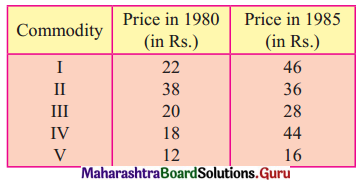Solution: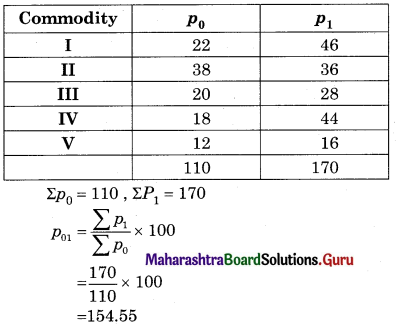Question 2.
Find the Quantity Index Number using Simple Aggregate Method.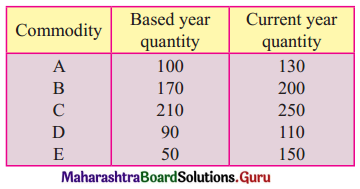Solution: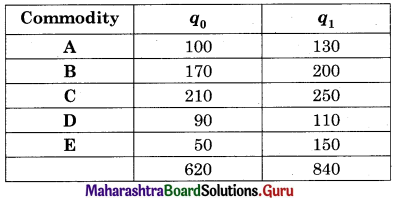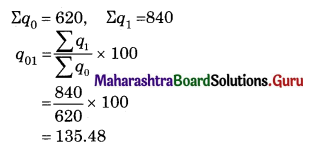Question 3.
Find the Value Index Number using Simple Aggregate Method.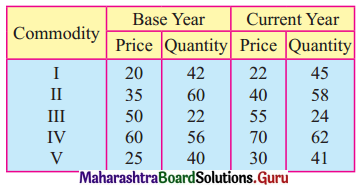Solution: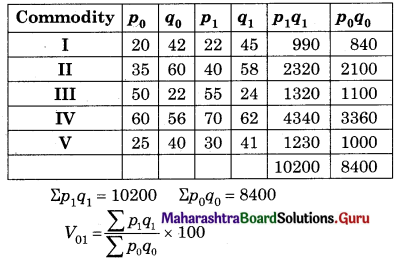= $$\frac{10200}{8400}$$ × 100
= 121.43

Question 4.
Find x if the Price Index Number using Simple Aggregate Method is 200.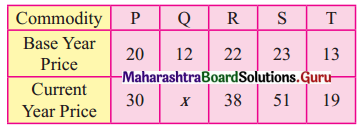Solution: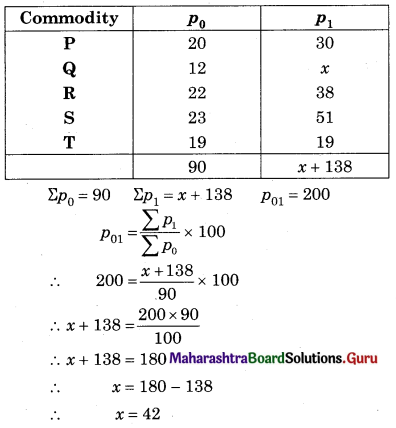Question 5.
Calculate Laspeyre’s and Paasche’s Price Index Number for the following data.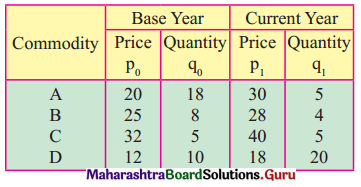Solution: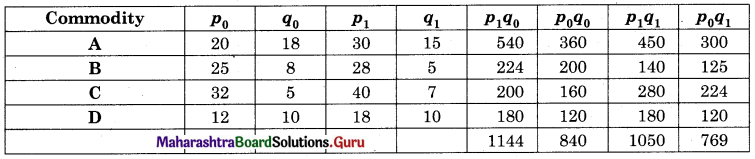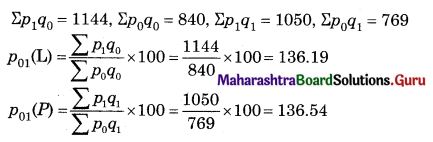Question 6.
Calculate Dorbish-Bowley’s Price Index Number for the following data.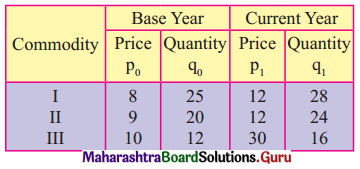Solution: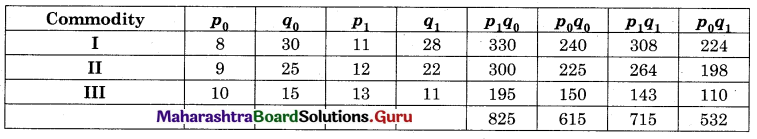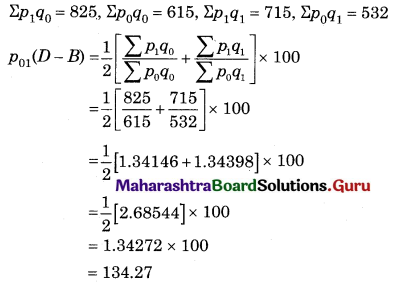Question 7.
Calculate Marshall-Edge worth’s Price Index Number for the following data.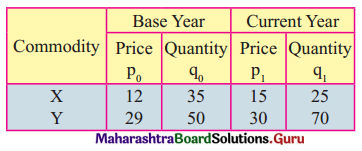Solution: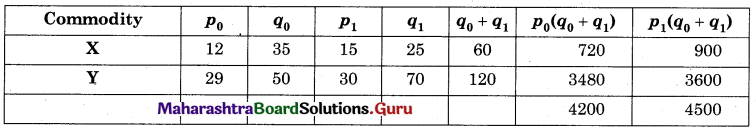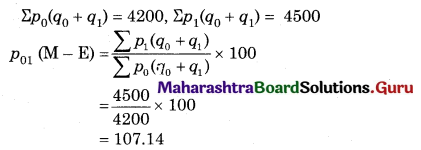Question 8.
Calculate Walsh’s Price Index Number for the following data.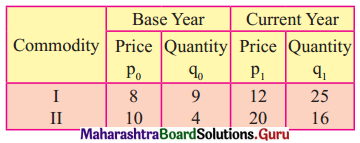Solution: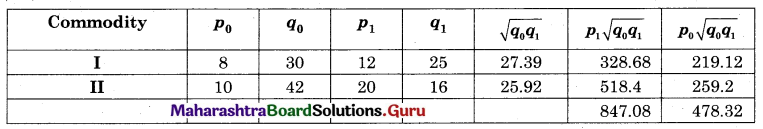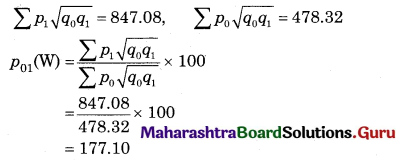Question 9.
Calculate Laspeyre’s Price Index Number for the following data.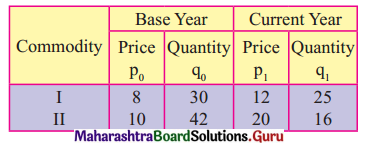Solution: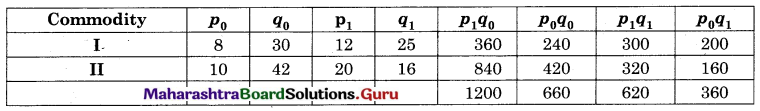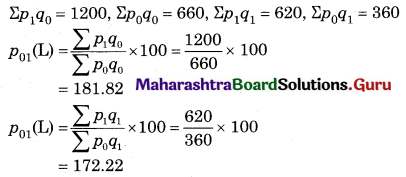Question 10.
Find x if Laseyre’s Price Index Number is same as Paasche’s Price Index Number for the following data.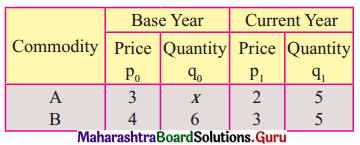Solution: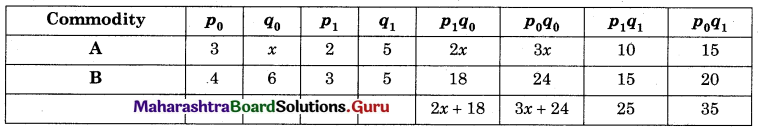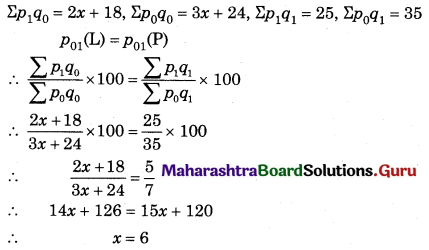Question 11.
Find x if Walsh’s Price Index Number is 150 for the following data.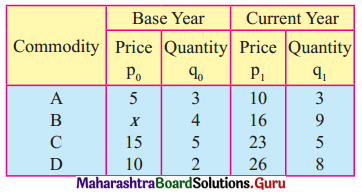Solution: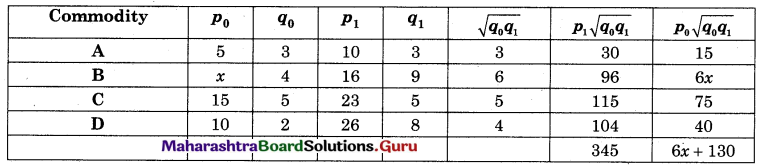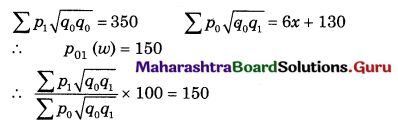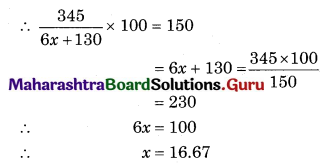Question 12.
Find x if Paasche’s Price Index Number is 140 for the following data.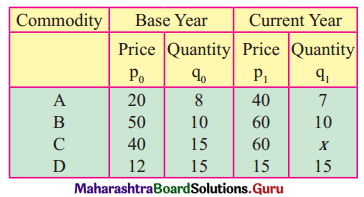Solution: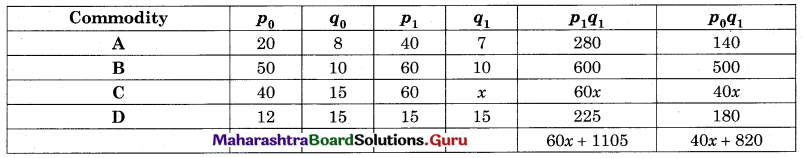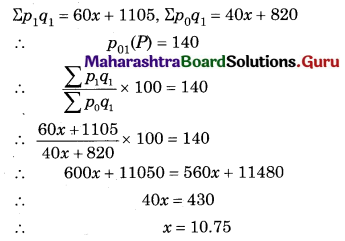Question 13.
Given that Laspeyre’s and Paasche’s Index Number are 25 and 16 respectively. Find Dorbish-Bowley’s and Fisher’s Price Index Number.
Solution: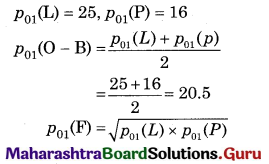= $$\sqrt{25 \times 16}$$
= 20Question 14.
If Laspeyre’s and Dorbish Price Index Number are 150.2 and 152.8 respectively, find Paasche’s rice Index Number.
Solution: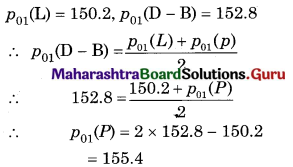Question 15.
If Σp0q0 = 120, Σp0q1 = 160, Σp1q1 = 140, and Σp1q0 = 200 find Laspeyre’s, Paasche’s, Dorbish-Bowley’s, and Marshall-Edgeworth’s Price Index Numbers.
Solution: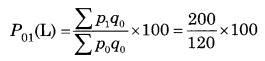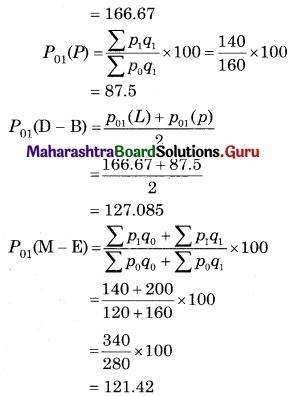Question 16.
Given that Σp0q0 = 130, Σp1q1 = 140, Σp0q1 = 160, and Σp1q0 = 200, find Laspeyare’s, Passche’s, Dorbish-Bowely’s and Mashall-Edegeworth’s Price Inbox Numbers.
Solution: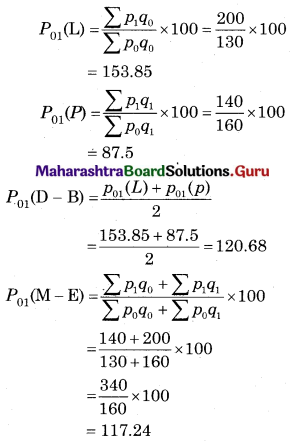Question 17.
Given that Σp1q1 = 300, Σp0q1 = 140, Σp0q0 = 120, and Marshall-Edegeworth’s Price Inbox Number is 120, find Laspeyre’s Price Index Number.
Solution:
p01(P) = $$\frac{\sum p_{1} q_{1}}{\sum p_{0} q_{1}} \times 100$$
= $$\frac{300}{320}$$ × 100
= 93.75

Question 18.
Calculate the cost of living number for the following data.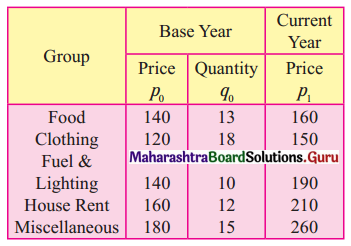Solution: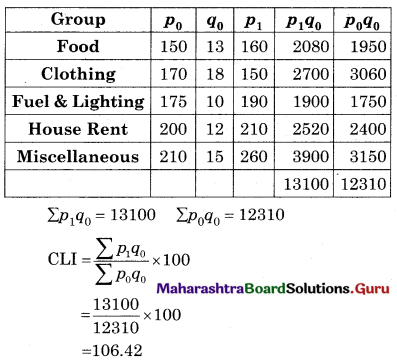Question 19.
Find the cost living index number by the weighted aggregate method.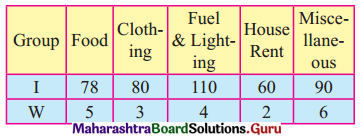Solution: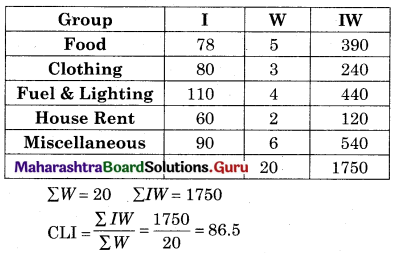Question 20.
Find the cost of living index number by Family Budget Method for the following data. Also, find the expenditure of a person in the year 2008 if his expenditure in the year 2005 was ₹ 10,000.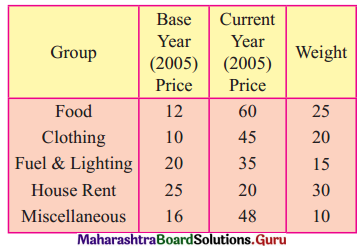Solution: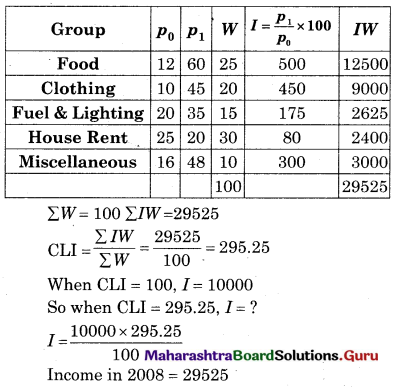Question 21.
Find x if cost of living index number is 193 for the following data.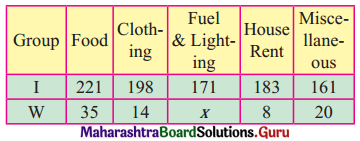Solution: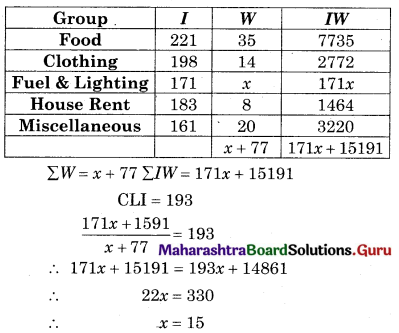Question 22.
The cost of living numbers for years 2000 and 2003 are 150 and 210 respectively. A person earns ₹ 13,500 per month in the year 2000. What should be his monthly earnings in the year 2003 in order to maintain the same standard of living?
Solution:
CLI (2000) = 150
CLI (2003) = 210
Income (2000) = 13500
Income (2003) = ?
Real Income = $$\frac{\text { Income }}{\mathrm{CLI}} \times 100$$
For 2000, Real Income = $$\frac{13500}{150} \times 100$$ = ₹ 9000
For 2003, Real Income = $$\frac{\text { Income }}{\mathrm{CLI}} \times 100$$
∴ 9000 = $$\frac{\text { Income }}{210} \times 100$$
∴ Income = $$\frac{9000 \times 210}{100}$$ = 18900
∴ Income in 2003 = ₹ 18900